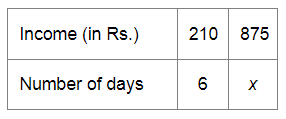# A worker is paid Rs 210 for 6 days work.`
Question:

A worker is paid Rs 210 for 6 days work. If his total income of the month is Rs 875, for how many days did he work?

Solution:

Let be the number of days for which the worker is paid Rs 875.Since the income of the worker and the number of working days are in direct variation, we have:

$\frac{210}{875}=\frac{6}{x}$

$\Rightarrow 210 \times x=875 \times 6$

$\Rightarrow x=\frac{875 \times 6}{210}$

$=\frac{5250}{210}$

$=25$

Thus, the required number of days is 25 .